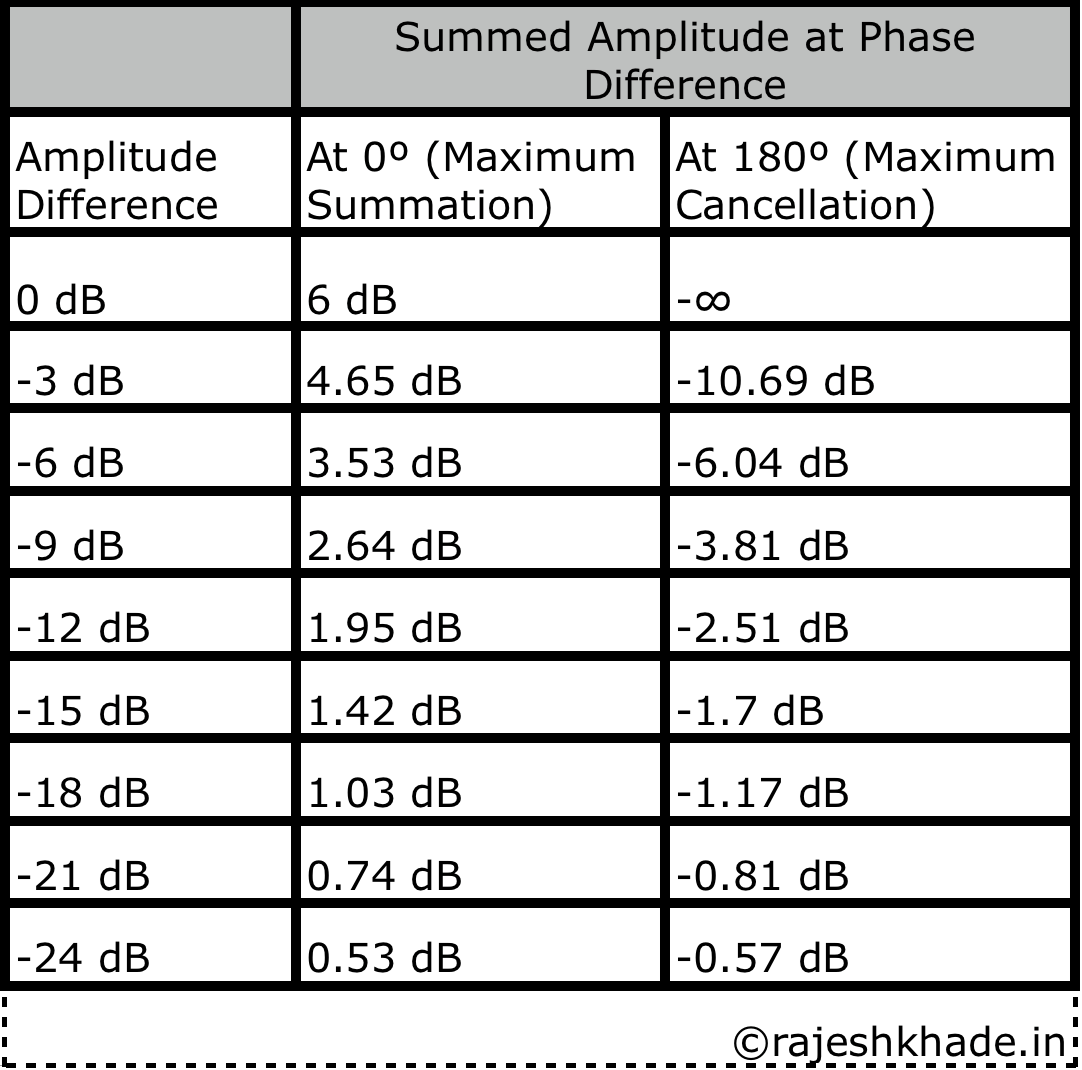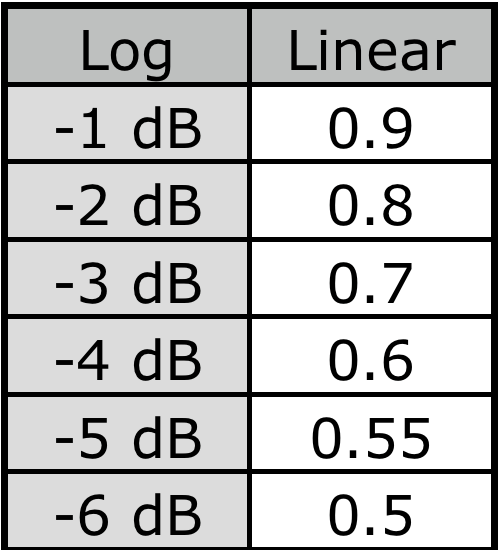#### Blog Post # 3 : Coupling vs Comb Filtering(Part 2) : Effects of varying Amplitude.

In part 1 of this series we saw the effects of phase differences on the interaction of two identical signals of same amplitude, leading to Coupling and Comb Filtering.

In this part 2 we will introduce another variable factor : ‘Amplitude’ and see how it affects the interaction. So, we will be seeing what happens when two identical signals with different amplitude and phase interact with each other.

To understand how amplitude affects the interaction of signals we will go through a bit of Mathematics.

This is a bit of a complicated topic as it requires an understanding of the maths being what is a dB and how does summation in dB work.

I’m gonna try to keep it as simple as I can and while doing so I’ll be skipping a few Why’s and How’s , for which you can either send me a Direct Message on my Facebook Page or may simply Google it.

1)The Mathematical formula we will be using is dB = 20 log (Output/Input).

2)The Mathematical formula for Summation : dB = 20 log (Signal 1 + Signal 2 / Signal 1)

#### The Difficult Part : Understanding dB addition!

Decibel abbreviated as dB is a relative value. So even when we say that a signal is 0 dB, we all know from our experience that 0 dB doesn’t mean absence of sound, it simply means that there is no level difference between the incoming and outgoing signal.

So, if Output signal = Input Signal = X.

The dB will be : 20 log (X/X) = 20 log (1) = 0 dB.

Now, if u remember Coupling from Part 1  : Two identical signals coming in phase causes a 6 dB gain! Where does this 6 dB gain come from :

Input Signal = X.

Output Signal = X + X = 2X.

So, dB = 20 log (2X/X) = 20 log (2) = 6 dB.

The purpose of doing these calculations is to study the summations of signals of different amplitude,so here are a few examples :

#### Example 1 :

We are adding two signals : S1 : X dB and a signal 2 that is 90% of S1.

So, S2 = 90/100 x S1 = 0.9S1 = 0.9X.

So the resultant Output Signal will be : Output : X + 0.9X = 1.9X.

Therefore, the summation of these signals will yield :

dB = 20 log (1.9x/x) = 20 log (1.9) = 5.57 dB.

#### Example 2 :

Similarly for signal 2 of 80% Strength of Signal 1. Output Signal = X +  0.8X = 1.8X.

Therefore the summation of these signals will yield :

dB = 20 log (1.8X/X) = 20 log (1.8) = 5.1 dB.

Similarly as we go on Calculating ,we will find :

Example 3 : For S2 = 60 % of S1 = 20 log (1.6) = 4.08 dB.

Example 4 : For S2 = 40% of S1  = 20 log (1.4) = 2.92 dB.

Example 5 : For S2 = 25% of S1  = 20 log (1.25) = 1.93 dB.

Example 6 : For S2 = 10% of S1  = 20 log (1.1) = 0.82 dB.

This was the difficult part, now for all practical purposes all we will remember is the linear scale ratio associated with particular dB value (approximating the decimal values of dB to the nearest whole number).2 : 6 dB

1.8 : 5 dB

1.6 : 4 dB

1.4 : 3 dB

1.25 : 2 dB

1.1 : 1 dB

1 : 0 dB.

Now, let’s see what happens when the output signal is less than the Input Signal (which is also representative of summation occurring in the subtraction area of phase cycle from greater than 120º to less than 240º .

#### Example 1 :

If the Summed Output Signal is 90% of Input :

dB = 20 log (0.9X/X) = 20 log (0.9) = 20 log (0.9) : -0.91 dB.

Example 2 : If the Summed Output Signal is 80 % of Input.

dB = 20 log (0.8X/X) = 20 log (0.8) = -1.93 dB.

Similarly we will find :

Example 3 : For 70 % of Original strength = 20 log (0.7) = -3 dB.

Example 4 : For 60 % of Original strength = 20 log (0.6) = -4.43 dB.

Example 5 : For 70 % of Original strength = 20 log (0.55) = -5.19 dB.

Example 6 : For 70 % of Original strength = 20 log (0.5) = -6.02 dB.

Considering example 6 : Notice how the input is double the output and hence the 6 dB, but as it’s the opposite situation of what we had earlier, it gets a negative sign and so -6 dB.

Again, simplifying these and only remembering the Linear scale Ratios and associated dB :0.9 : -1 dB

0.8 : -2 dB

0.7 : -3 dB

0.6 : -4 dB

0.55 : – 5 dB

0.5 : -6 dB

#### To summarize all calculations : For On Field Reference :

All of this data is made available in a single slide, showing the relationship between Linear Ratio and Log Scale by the amazing  El Magu : Mauricio Ramirez, Senior Technical Seminar Instructor, Meyer Sound!

A big thanks to Mauricio for the slide!

#### Simplified and Practical Application of Data :

Now that we know how the above data has been derived, we will put it to some practical use.

Example 1 : Summation of 2 identical signals of 0 dB & -3 dB.

So, we will identify their respective linear scale values and do linear mathematics :

0 dB : 1 & -3 dB : 0.7.

When Phase Difference = 0º : Maximum Summation : 1 + 0.7 = 1.7 which corresponds to a dB value between 4 dB and 5 dB.

When Phase Difference = 180º : Maximum Cancellation : 1 – 0.7 = 0.3 which corresponds to a dB value of -10 dB.

Verifying Logarithmically : a)20 log (1.7) = 4.6 dB. b)20 log (0.3) = -10.45 dB.

Example 2 : Summation of 2 identical signals of 0 dB and -9 dB.

0 dB : 1 & -9 db : 0.35.

When Phase Difference = 0º : Maximum Summation : 1 + 0.35 = 1.35 : corresponds to a dB value between 2 dB and 3 dB.

When Phase Difference = 180º : Maximum Cancellation : 1 – 0.35 = 0.65 : corresponds to a dB value between -4 dB & -3 dB.

Verifying Logarithmically : a)20 log (1.35) = 2.6 dB. b)20 log (0.65) = -3.74 dB.

To summarise all of these Calculations :

I have made this above slide showing the maximum summation and maximum cancellation when two identical signals with an amplitude difference interact with each other.

I’m gonna conclude this part here as this data is a lot to digest. So, go through this article and try out different combinations to get used to estimating approximate dB when two signals interact.

In part 3 we will use all of this data we have learnt in Part 1 and Part 2, to see the interaction on an analyser!

Till then,